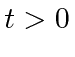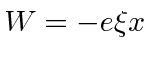## Homework Problems

1. A hydrogen atom is placed in an electric field which is uniform in space and turns on atthen decays exponentially. That is,forandfor. What is the probability that, as, the hydrogen atom has made a transition to thestate?

2. A one dimensional harmonic oscillator is in its ground state. It is subjected to the additional potentialfor a a time interval. Calculate the probability to make a transition to the first excited state (in first order). Now calculate the probability to make a transition to the second excited state. You will need to calculate to second order.

Jim Branson 2013-04-22